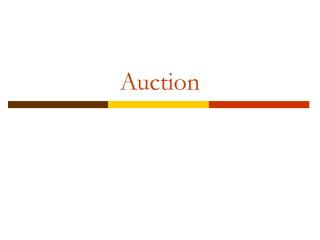DownloadDownload PresentationAuction

# Auction

Télécharger la présentation## Auction

- - - - - - - - - - - - - - - - - - - - - - - - - - - E N D - - - - - - - - - - - - - - - - - - - - - - - - - - -
##### Presentation Transcript

1. Auction

2. Types of Auction • Open outcry • English (ascending) auction • Dutch (descending) auction • Sealed bid • First-price • Second-price (Vickrey) • Equivalence in these auctions? • Common-value auction • Private-value auction

3. The winner’s curse When one places a bid (say \$b) in a common-value auction and is accepted, the value to the seller’s must be less than \$b (0-b). Otherwise the item will not be sold. The common value is probably about \$b/2 given the average is an unbiased estimate. (Note: everyone’s estimate is private information.)

4. Auction and incomplete information • In the private-value auction, every bidder has private information about her evaluation on the item sold. • Ex: vi~U(0,1),i.i.d. bidders are risk neutral

5. Bidding Strategy • First-price • Bidder with higher valuation will bid higher • Given your evaluation is highest, expectation of the 2nd highest valuation • Ex: if one’s valuation is x, then bids (n-1)x/n

6. Second-price • The bidder will bid truthfully her evaluation on the item. • Truthfully bidding is a weakly dominant strategy. • Revenue Equivalence Principle • The seller will collect the same amount from either 1st-price of 2nd-price auction. • Assumptions of independence, identical distribution, risk neutral matters.

7. All-pay auction • Every bidder pays a sunk (unrecoverable) cost of her bid, and the one with the highest bid wins the item. • Ex: Olympic games, political lobbying, R&D races • Equilibrium bidding strategy must be a mixed strategy. • Consider a common-value all-pay auction with prize worth 1.

8. Bid x in (0,1) will be continuous • Let P(x) be the equilibrium cdf, the probability one’s bid is not higher than x. • Indifference principle • Bidding x: [P(x)]n-1-x • Bidding 0: 0 • → P(x)=x 1/(n-1) • When n=2, P(x)=x • A uniform distribution for pdf of x

9. When n=3, P(x)=x1/2 • X=1/4, P(x)=1/2, ½ of chance the bid will be lower than 1/4 • As n increases, it’s more likely to bid lower. • Expected payment is 1/n for everyone.